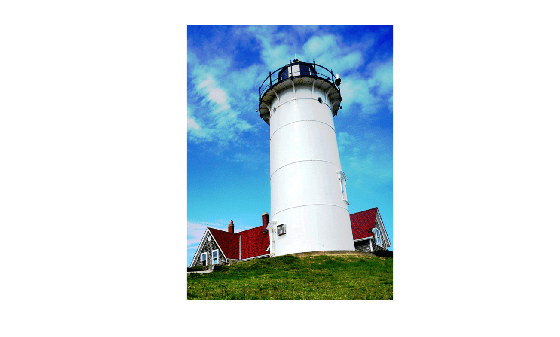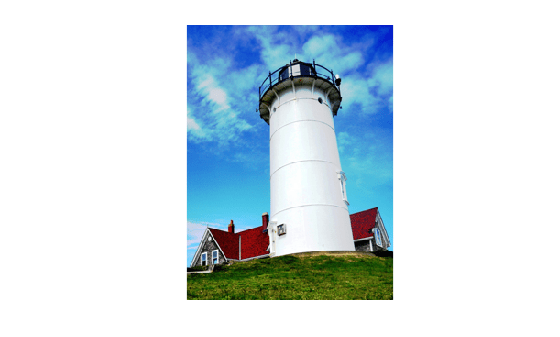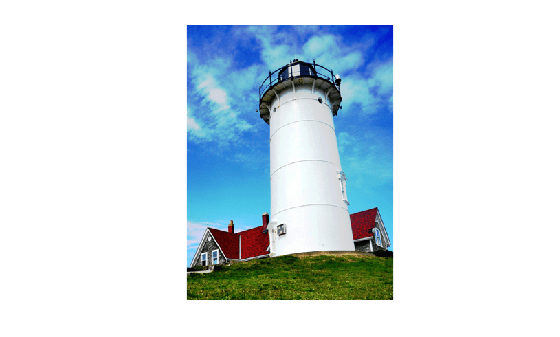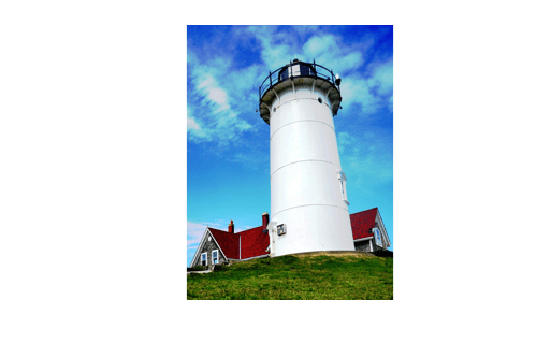# Resize Image and Preserve Aspect Ratio

This example shows how to resize an image while maintaining the ratio of width to height. This example covers two common situations:

1. You want to resize the image and specify the exact height or width.

2. You want to resize an image to be as large as possible without the width and height exceeding a maximum value.

Start by reading and displaying an image.

```I = imread("lighthouse.png"); imshow(I)```Get the size of the image. The aspect ratio of this image is 3:4, meaning that the width is 3/4 of the height.

`[heightI,widthI,~] = size(I)`
```heightI = 640 ```
```widthI = 480 ```

### Resize Image to Specified Height

Specify the desired height of the image.

`targetHeight = 300;`

Resize the image, specifying the output size of the image. Because the second element of `targetSize` is NaN, `imresize` automatically calculates the number of rows needed to preserve the aspect ratio.

```targetSize = [targetHeight NaN]; J = imresize(I,targetSize); [h,w,~] = size(J)```
```h = 300 ```
```w = 225 ```
`imshow(J)`### Resize Image to Specified Width

Specify the desired width of the image.

`targetWidth = 300;`

Resize the image, specifying the output size of the image. Because the first element of `targetSize` is NaN, `imresize` automatically calculates the number of columns needed to preserve the aspect ratio.

```targetSize = [NaN targetWidth]; K = imresize(I,targetSize); [h,w,~] = size(K)```
```h = 400 ```
```w = 300 ```
`imshow(K)`### Resize Image Within Maximum Size

You can resize an image to be as large as possible without the width and height exceeding a maximum value.

Specify the maximum dimensions of the image.

```maxHeight = 256; maxWidth = 256;```

Determine which dimension requires a larger scaling factor.

`scaleWidth = widthI/maxWidth`
```scaleWidth = 1.8750 ```
`scaleHeight = heightI/maxHeight`
```scaleHeight = 2.5000 ```

Select the output size based on whether the height or width requires a greater scale factor to fit within the maximum image dimensions. If the height requires a greater scale factor, then specify the target height as `maxHeight`. Conversely, if the width requires a greater scale factor, then specify the target width as `maxWidth`. Let `imresize` automatically calculate the size of the other dimension to preserve the aspect ratio.

```if scaleHeight>scaleWidth targetSize = [maxHeight NaN]; else targetSize = [NaN maxWidth]; end```

Resize the image, specifying the output size of the image.

```L = imresize(I,targetSize); [h,w,~] = size(L)```
```h = 256 ```
```w = 192 ```
`imshow(L)`